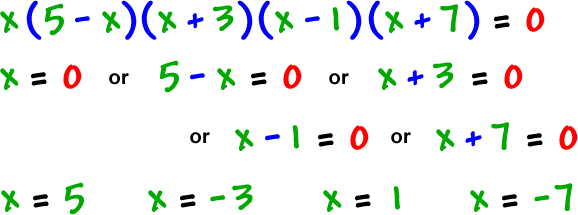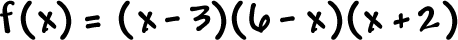This one has a twist...

Find the real zeros ofthen draw a rough sketch of the graph:

Do you see the twist?  It's the (5 - x) guy!

So, what's his basic shape?What are the real zeros?real zeros: -7, -3, 0, 1, 5

TRY IT:

Find the real zeros of the functions and draw a rough sketch of the graph: